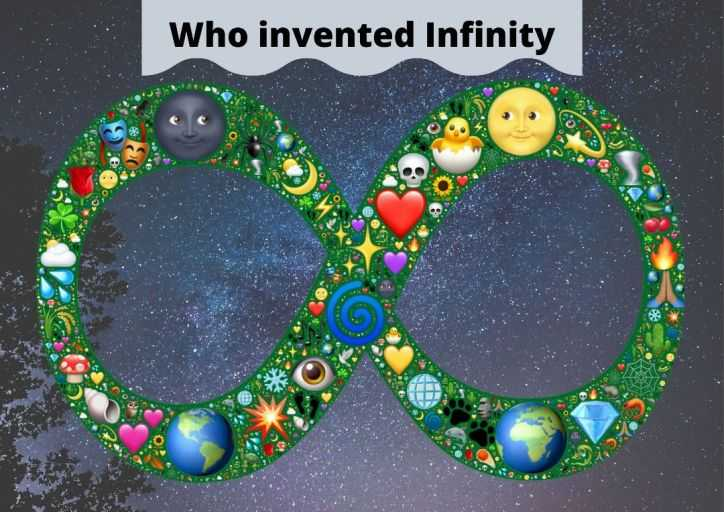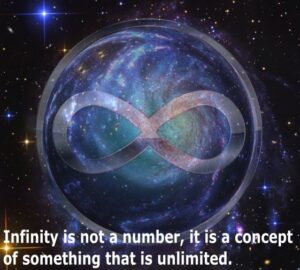# Who invented Infinity: History and InformationInfinity is the concept of something that is unlimited, endless, unbound, or something is bigger than any natural number in mathematics. The concept and nature of infinity have been mentioned by Greeks and Indians since ancient times.

Though the concept and idea of infinity were known since ancient times. But the common symbol of infinity “∞” was first time used in 1655 by the English clergyman and mathematician John Wallis.

Here we are going to look at the history of the invention of infinity but before that, we will look at what is the meaning of infinity.

## What is infinity?

Infinity is not a number, it is a concept of something that is unlimited. You can say ‘infinity’ is just a name for a specific idea. Infinity is not the biggest number though there is no number bigger than infinity. And thus it is the meaning of infinity.

It seems like a contradiction but it is not the biggest number because it is not a number. In fact, there is no biggest number in the number system (so simple…lol).

According to mathematicians, there are two types of infinity sets

1. Countable sets of infinity
2. Uncountable sets of infinity

Countable infinity sets are those which we can count as a natural number until reached infinity. Like a set of natural numbers (1, 2, 3, 4….), and other mathematical infinity. Whereas the uncountable infinity sets are those which we can not count; such as ‘space’, ‘direction’, ‘time’, etc.Meaning of Infinity

## History of invention of the Infinity

In the late 17th century development of infinitesimal calculus was started especially by Isaac Newton and Gottfried Wilhelm Leibniz. Other mathematicians also started work on infinite series but they struggled in the calculation. Mathematicians were unable to find out, whether infinity is a number or quantity or magnitude and if so, then how to represent it.

In ancient times people were understanding the nature of infinity but they have given only philosophical concepts. In philosophy infinity means “impossible to reach”. Whereas modern mathematics gives a precise way to understand it.

So how the infinity got to know us, is really invented or discovered by mathematicians? And if invented then who invented infinity?

#### Ancient Greek

A Greek philosopher Anaximander (c. 610 – c. 546 BC) used the word “Apeiron” in his philosophy. The meaning of the Aperion in philosophy is ‘unbound’, ‘indefinite’, ‘without limit’, and ‘without end’. It was the first recorded concept of infinity that has a philosophical meaning.

Also, the Greek philosopher Aristotle (384 – 322 BC) shared a notion of infinity and distinguished potential infinity from actual infinity.

Aristotle states that “It is always possible to think of a larger number: for the number of times a magnitude can be bisected is infinite. Hence the infinite is potential, never actual; the number of parts that can be taken always surpasses any assigned number”.

#### Ancient Indian

An Indian mathematician Surya Prajnapti (4th – 3rd century BCE) categorized all numbers into 3 sets. enumerable, innumerable, and infinite. These sets are further divided into:

• Enumerable: lowest, intermediate, and highest
• Innumerable: nearly innumerable, truly innumerable, and innumerably innumerable
• Infinite: nearly infinite, truly infinite, infinitely infinite

Indian Jains were the first who defined the different ‘types’ of infinities in their concepts. They were the first who said that all infinities are not the same. Jains also classified infinity into different dimensions:

• Infinity in length (one dimension)
• Infinity in the area (two dimensions)
• Infinity in volume (three dimensions)
• Infinite perpetually (infinite number of dimensions)

Scripture of the Hinduism Yajurveda (c. 4th – 3rd century BC) states that “if we remove a part from infinity or add a part to infinity, still what remains is infinity”.

#### Infinity in 17th century

In modern mathematics like in the 17th century, European mathematicians started using the concept of infinity in their mathematical works. John Wallis gave the symbol for infinity ‘∞’ in 1655

A German mathematician Georg Cantor (1845-1918) became the first one who used infinity in his mathematical framework clearly. Cantor defined the existence of ‘infinity in infinities’ and also gave a theorem of ‘infinite set’ in calculus.

### Conclusion for who invented infinity

Human has the ability to think and many of them must have thought about the existence of infinity before we could find out. As we know infinity is not even a number then how anyone can invent it. So if you have a question for who invented infinity? Then according to me, it was not invented, it was just discovered and formulated by many people.

People gave the concept or idea for it, as it was always present since the universe got into existence. Ancient Greek, Indian, and European all of them just clarified it with different facts that were already present. Many scientists contributed to formulating it, to using it properly in the area of mathematics, physics, computer, and many more.

### Important points to know about Infinity

1. The symbol of Infinity is also known as ‘Lemniscate’ and it has been taken from the Latin word “Lemniscus”, which means “Ribbon”.
2. Some mathematical undefined values for infinity:-
• ∞/∞
• 0 × ∞
• ∞ × ∞
• 0/∞
• 0
• ∞ – ∞

These values related to infinity in mathematics do not make any sense and are known as undefined values. Scientists are continuously searching for the mystery of infinity considering the whole universe. Some mysteries have been discovered/invented but there is still a lot we have to search for what is infinity

1.